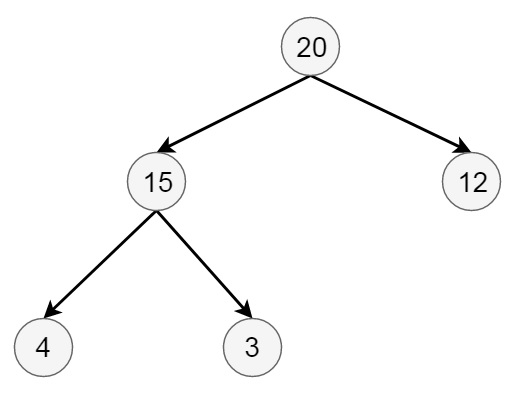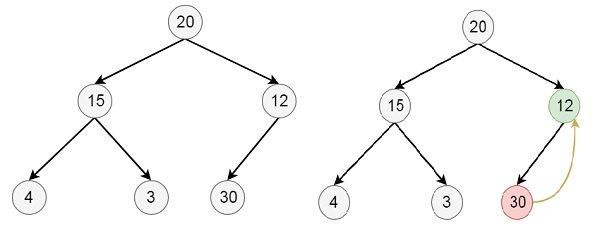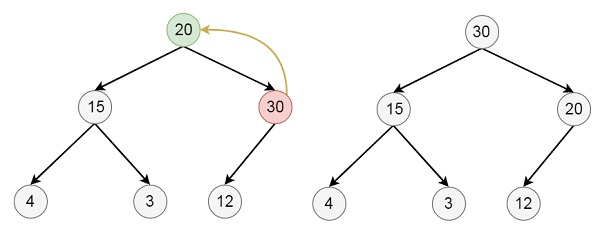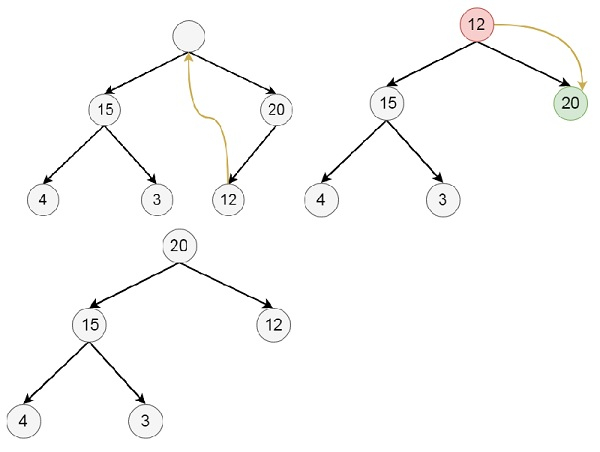# Insertion and Deletion in Heaps in Data Sturcture

Data StructureAnalysis of AlgorithmsAlgorithms

Here we will see how to insert and delete elements from binary heap data structures. Suppose the initial tree is like below −## Insertion Algorithm

insert(heap, n, item):
Begin
if heap is full, then exit
else
n := n + 1
for i := n, i > 1, set i := i / 2 in each iteration, do
if item <= heap[i/2], then break
heap[i] = heap[i/2]
done
end if
heap[i] := item
End

## Example

Suppose we want to insert 30 into the heap −## Deletion Algorithm

delete(heap, n):
Begin
if heap is empty, then exit
else
item := heap
last := heap[n]
n := n – 1
for i := 1, j := 2, j <= n, set i := j and j := j * 2, do
if j < n, then
if heap[j] < heap[j + 1], then j := j + 1
end if
if last >= heap[j], then break
heap[i] := heap[j]
done
end if
heap[i] := last
End

## Example

Suppose we want to delete 30 from the final heap −